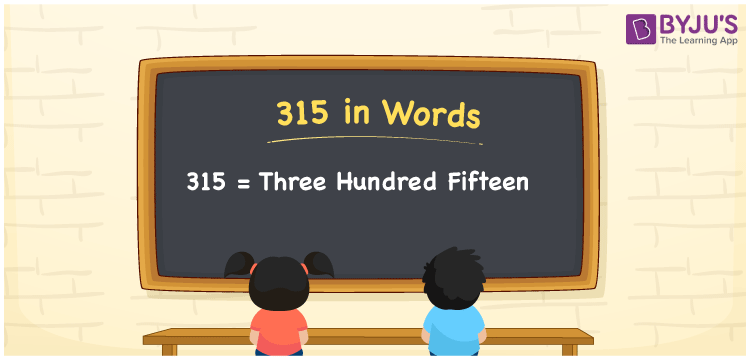# 315 in Words

315 in words is written as Three hundred fifteen. In both the International System of Numerals and the Indian System of Numerals, 315 is written as Three hundred fifteen. The number 315 is a Cardinal Number as it represents some quantity. For example, “there are 315 chairs in the room”.

 315 in Words Three hundred fifteen Three hundred fifteen in Number 315

## 315 in English Words

We write 315 in English Words using the letters of the English alphabet. Therefore, we read 315 in English as “Three hundred fifteen.”## How to Write 315 in Words?

To write 315 in words, we shall use the place value chart. In the place value chart, write 3 in the hundreds, 1 in the tens, and 5 in the ones, respectively. Now let us make a place value chart to write the number 315 in words.

 Hundreds Tens Ones 3 1 5

Thus, we can write the expanded form as

3 × Hundred + 1 × Ten + 5 × One

= 3 × 100 + 1 × 10 + 5 × 1

= 300 + 10 + 5

= 315

= Three hundred fifteen.

315 is a natural number, the successor of 314 and the predecessor of 316.

315 in words – Three hundred fifteen

• Is 315 an odd number? – Yes
• Is 315 an even number? – No
• Is 315 a perfect square number? – No
• Is 315 a perfect cube number? – No
• Is 315 a prime number? – No
• Is 315 a composite number? – Yes

## Frequently Asked Questions on 315 in Words

Q1

### How to write 315 in words?

315 in words is written as Three hundred fifteen.
Q2

### How to write 315 in the International and Indian System of Numerals?

In both, the system of numerals, 315 in words, is written as Three hundred fifteen.
Q3

### What is the preceding number of 315?

The number that precedes 315 is 314.